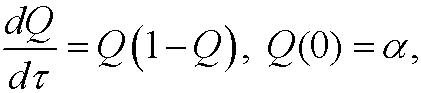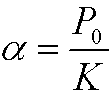# 9. Some population models.

We will here formulate some simple models for poplation dynamics in a closed ecosystem. Such models play an important role when it comes to understanding the factors that contributes to increase in population. Let P=P(t) denote the size of the population at time t and P0denote the size of the population at time t=0.

The most simple model is known as Malthus’ model and it says that the increase in population is proportional to the size of the population, that iswhere r is a constant speed of growth. This model predicts that the population will increase exponentially, that isThis would lead to a population explosion. Is this model reasonable? As the population grows, the amount of food, living space and natural resources will limit the growth. We should therefore correct Malthus’ model with a limiting term. The growth not only depend on the size of the population but also on how far it is from its upper limit. We must correct with a term that makes the growth decrease and becomes zero when we reach this upper limit K. We have thus reached the logistic model (also known as Verhulst’s population model)where K is a constant that can be interpreted as the largest amount of individuals that an ecosystem kan nourish (the so called carrying capacity).
Now we scale this model. We introduce a dimensionless timeand a dimensionless populationWe can now write the logistic equation in these dimensionless variablesthat iswhereis dimensionless. This differential equation can for instance be solved by separation of variablesWe easily realize thatthat is<>The constant solution P=K is called attractor and has the property that independent of the size of the initial population, the size of the population will tend to K as the time goes to infinity. Attractors and related phenomena of dynamical systems will be examined in chapter IX.

www.larserikpersson.se